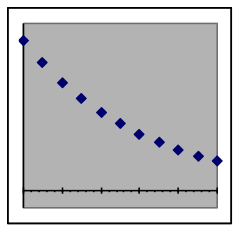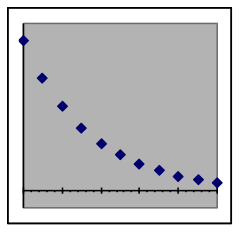$$\newcommand{\id}{\mathrm{id}}$$ $$\newcommand{\Span}{\mathrm{span}}$$ $$\newcommand{\kernel}{\mathrm{null}\,}$$ $$\newcommand{\range}{\mathrm{range}\,}$$ $$\newcommand{\RealPart}{\mathrm{Re}}$$ $$\newcommand{\ImaginaryPart}{\mathrm{Im}}$$ $$\newcommand{\Argument}{\mathrm{Arg}}$$ $$\newcommand{\norm}{\| #1 \|}$$ $$\newcommand{\inner}{\langle #1, #2 \rangle}$$ $$\newcommand{\Span}{\mathrm{span}}$$

# 22.2: J1.02: Section 1 Part 1

$$\newcommand{\vecs}{\overset { \rightharpoonup} {\mathbf{#1}} }$$ $$\newcommand{\vecd}{\overset{-\!-\!\rightharpoonup}{\vphantom{a}\smash {#1}}}$$$$\newcommand{\id}{\mathrm{id}}$$ $$\newcommand{\Span}{\mathrm{span}}$$ $$\newcommand{\kernel}{\mathrm{null}\,}$$ $$\newcommand{\range}{\mathrm{range}\,}$$ $$\newcommand{\RealPart}{\mathrm{Re}}$$ $$\newcommand{\ImaginaryPart}{\mathrm{Im}}$$ $$\newcommand{\Argument}{\mathrm{Arg}}$$ $$\newcommand{\norm}{\| #1 \|}$$ $$\newcommand{\inner}{\langle #1, #2 \rangle}$$ $$\newcommand{\Span}{\mathrm{span}}$$ $$\newcommand{\id}{\mathrm{id}}$$ $$\newcommand{\Span}{\mathrm{span}}$$ $$\newcommand{\kernel}{\mathrm{null}\,}$$ $$\newcommand{\range}{\mathrm{range}\,}$$ $$\newcommand{\RealPart}{\mathrm{Re}}$$ $$\newcommand{\ImaginaryPart}{\mathrm{Im}}$$ $$\newcommand{\Argument}{\mathrm{Arg}}$$ $$\newcommand{\norm}{\| #1 \|}$$ $$\newcommand{\inner}{\langle #1, #2 \rangle}$$ $$\newcommand{\Span}{\mathrm{span}}$$

## Section 1: Processes with constant-percentage growth/decay rates—exponential models

In situations where the cause of change in a quantity is the amount of that quantity that is currently present, the output variable changes by the same percentage for each equal step in the input variable. Such situations have an exponential model formula, which means that the input variable is used as an exponent in the formula. Accumulation of compound interest in a bank account is an example of exponential growth, and radioactive decay is an example of exponential decay. Both are modeled by exponential formulas, with the difference between growth and decay depending simply on whether the change rate is positive or negative.

 Relationship of different exponential models to the x-axisExponential growth Faster growth rate Exponential decay Faster decay rate

Examples of exponential formulas:$y=275\cdot{{(1+0.05)}^{x}}\\y=65.08\cdot{{(1-0.03)}^{x}}\\y=-6400\cdot{{(1-0.25)}^{x}}\\y=0.00836\cdot{{(1+0.21)}^{x}}$

Since any number raised to the power of zero equals exactly 1, the y-intercept of an exponential formula (i.e., the formula y value when x equals zero) will be just the multiplier term (such as 275, 65.08, 6400, and 0.00836 above). This intercept is one parameter of the exponential model, and reflects the starting value for processes that start at x=0.

The other parameter reflects the rate at which the value of the formula will change. The simplest way of expressing it is to use the desired rate to make an appropriate base for the input parameter x as an exponent. This is the role of “1+0.05”, “1-0.03”, “1-0.25”, and “1+0.21” in the examples above. The growth rates of 0.05, –0.03, –0.25, and 0.21 could instead be expressed as 5%, –3%, –25%, and 21%.

A negative growth rate is actually a decay rate, since it will result in values closer to zero as x becomes larger. When the growth rate is negative, the base of the exponent will be a number that is less than 1 but greater than 0. Thus the base for a –0.03 growth rate (that is, a 3% decay rate) would be 0.97, and the base for a –0.25 growth rate (i.e., a 25% decay rate) would be 0.75.

Warning: An exponential decay rate can never be more than 100% (that is, have a growth rate of less than –1.0). Such a rate would not correspond to a realistic situation, and would make the base of the exponent a negative number. Spreadsheets will give error messages when negative numbers are used as a base in calculations with decimal exponents (unless the exponent is exactly a whole number).

Exponential models include all$y=a\cdot{{b}^{x}}$forms such as:$y=InitialAmount\cdot{{(1+GrowthRate)}^{x}}$ or$y=InitialAmount\cdot{{(1-DecayRate)}^{x}}$

InitialAmount is the y value at x = 0

Note that some data may have inputs starting at non-zero values (e.g., 1975), in which case it will usually make the model simpler if the inputs are redefined to start at zero (e.g., as “years since 1975”) or if the model is modified to adjust for a non-zero starting value, as is illustrated in a later section.

GrowthRate is the relative increase in y when x increases by 1

The growth rate is usually expressed in formulas as a decimal number but is also often described as a percentage (e.g., 0.05 is the decimal representing 5%). For exponential decay, the growth rate is negative but must be between zero and minus one (that is, between 0% and –100%). A growth rate of zero would result in the output not changing from its initial value, since 1 raised to any power is still 1.

Often the formula will have the growth rate already combined with the 1, so that the growth term becomes (1.05)x or (105%)x. For negative rates, this term will be less than 1, such as (0.95)x or (95%)x.

Percentages must be changed to decimals when computing with them. Most spreadsheet programs will do this automatically if the percentage number is followed by a % character.